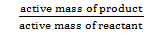# Free Energy Change And Net Useful Work

Work other than pressure volume work (PΔV) is net useful work. So, total work of a process is,

W = Wnet + PΔV

Wnet = W – PΔV

From Gibb’s helmuntz equation,

ΔG = H – TΔS ……………. (i)

We have,

H = E + PΔV
S = Q /T

TΔS = Q

Putting the values of H and TS in eqn (i), we get
ΔG = ΔE + PΔV – Q

From first law of thermodynamics
Q = ΔE + W

Putting the value of Q in above enq we get,
G = Δ E + PΔV – ΔE – W
G = PΔV – W
G = – (W – PΔV)
G = – Wnet
Wnet is – ΔG
Thus, the net useful work is the amount of decrease in free energy of a system under constant temperature and pressure.

Relation between free energy change and cell potential

The net work done by galvanic cell in carrying charge is

W = charge × potential difference = nf Ecell

We have,
ΔG = -Wnet
ΔG = -nfEcell

Relation between G and equilibrium constant

The variation of G is given by Nernst equation as,
G = ΔG + RT in Q

Where, Q = reaction quotientWhere, the reaction is at equilibrium
g = k (equilibrium constant)
ΔG = O
O = G° + RT in k
ΔG° = -RT InK
ΔG = -2.303 RT logK

The overall reaction for corrosion of iron by oxygen is,

4Fe (s) + 3O2 (g) → 2Fe2O3 (s) Rust

Calculate the free energy change and equilibrium constant for this reaction at 250°c.

Given,

ΔS° and ΔH° = -543 JK-1 and -1652 KJ mol-1
= -1652000 Jmol-1

Δ G° = Δ H° – TΔS°
= 1652000 – 298 × (-543)
= -1490186 J

Again,

ΔG° = -RTInK
= -2.303 RTlogK
1490186 = – 2.303 × 8.314 × 298 × log K
logK = 261.17
K = 10261.17
K = 1.48 × 10261.17

Calculate the free energy at standard condition for galvanic cell having following cell reaction.

2Al (s) + 3 Cu ++ (aq) → 2Al+++ (aq) + 3Cu (s)

E° Cu++/Cu = 0.34 V → E° Al+++/A1 = -1.66V

So, the standard cell potential,

E°cell = E°cathode – E°anode

= E°cu++/cu – E°Al+++/A1
= 0.4 – (-1.66)
= 2V

Again, Δ G = -nfEcell
= – 6 × 96500 × 2
= 1.158 × 106J